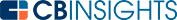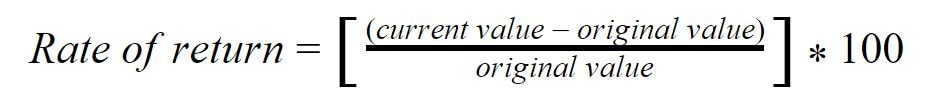# What is the Rate of Return Formula?The rate of return formula calculates the total net profit an investor makes from an asset. It is calculated and shown as a percentage of the total initial cost of the asset. Essentially, you are calculating the percentage change from the beginning to the end of an investment.

## Rate of return definition

Rate of return (RoR) is the calculated net returns made from an investment of any class. It considers the total returns, so incorporates dividends, interest payments, and capital gains.

The concept is used by investors, and it can also be used by businesses and organizations to determine the returns of a range of asset classes including stocks and shares, bonds, real estate, business investment, art, and bullion holdings.

There are different classes of RoR, each taking different factors into account during their calculation:

## Simple rate of return

The simple rate of return is the total net income earned from an asset, divided by the total initial investment. This gives returns as a percentage of an investment, but it does not take factors such as inflation into account.

It is also referred to as the growth rate or return on investment (ROI), as well as the nominal, unadjusted, or accounting rate of return.

The formula for calculating simple RoR is:For example, if an investor buys \$1,000 of shares and sells them two years later for \$1,100, their simple rate of return is equal to 10%.

## Real rate of return

Also called the inflation adjusted rate of return, the real rate of return adjusts returns for the effects of inflation.

The nominal value assumes that money holds its value over time. Inflation rates are determined by the amount of goods that money will buy compared to the year before and is a more reliable measure of the value of money. Using the inflation adjusted rate of return means that you can calculate returns compared to how much your initial investment would have been worth if you had held onto the cash.

The Consumer Price Index (CPI) is the most widely used measure of inflation, although investors and businesses may use other given inflation rates or a basic, annualized rate.

It is calculated using the formula:A simple and rough calculation can be made by subtracting the inflation rate from the nominal rate of return.

For example, if an investment returned \$1,100 over a 12-month period and the investor determines the inflation rate to be 2% during that period, they could calculate the real rate of return with the following equation.Using the simplified version of this equation, we would get a real RoR of 8%.

## Annualized rate of return

The compound annual growth rate (COGR) is also known as the annualized rate of return. It calculates the return on investment per year and can be calculated for any number of years.

The annualized rate of return can be calculated with the following formula:For example, the annualized rate of return on an investment of \$1,000 that returns \$1,100 after three years can be calculated using the following equation:Using this formula, we would get an annualized rate of return of 3.2%.

## Internal RoR

Internal rate of return (IRR) also takes into account the value of time and calculates returns against expected returns. It is therefore considered a value of performance against expectations. A positive IRR means that an investment has performed above expectations, while a negative IRR means that it has underperformed.

The internal rate of return is typically used by businesses to determine which, of several investment opportunities or business projects, will yield the greatest investment returns.

The rate of return is a method of displaying the return of an investment. It is expressed as a percentage of the initial investment and it can be positive or negative. Variants include the nominal rate of return, which does not consider inflation, and the real rate of return, which is inflation adjusted.

You may also like# www.cbinsights.com